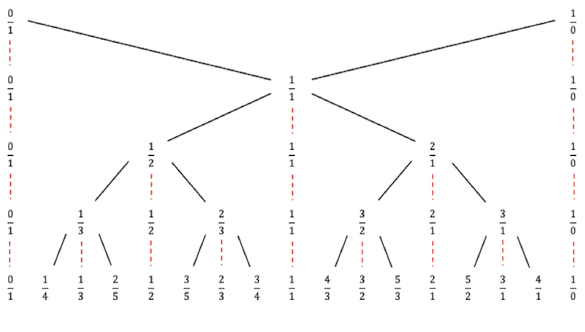# Stern-Brocot Tree

Stern-Brocot tree is a tree data structure whose vertices correspond to the set of non-negative rational numbers. Thus, this tree provides a very elegant way for constructing the set of fractions `m/n`, where `m` and `n` are relatively prime. To construct the tree, the basic idea is to start with two fractions (`0/1`, `1/0`) and then repeat the following operation:

Insert (m+m’)/(n+n’) between two adjacent fractions m/n and m’/n’

The first step gives us the entry `1/1` between `0/1` and `1/0`. Similarly, the 2nd step gives us two more: `0/1`, `1/2`, `1/1`, `2/1`, `1/0`.

Continuing on like this results in an infinite binary search tree which preserves the usual ordering of rational numbers.

The figure below shows the 1st 4 levels of the Stern-Brocot tree.The first 4 levels of Stern-Brocot Tree

## Finding the Path to k in Stern-Brocot Tree

The path from the root of the tree to a number `k` in the Stern-Brocot tree can be found using binary search. At each node, `k` will either be in the left half of the tree, or the right half. We continue down the left or right subtree until we finally find `k`.

• Initialize the left fraction `L` to `0/1` and right fraction `R` to `1/0`
• Repeat the following until `k` is found:
• Compute the mediant `M` (which is `(m+m')/(n+n')` )
• If `(k>M)`, then `k` is in the right half of the tree. `L:=M` and continue.
• Else If `(M>k)`, then `k` is in the left half of the tree. `R:=M` and continue.
• Else `k=M`, terminate search.

## Implementation

There’s a couple of things to tackle in our implementation. First, I need an easy way to represent fractions, so I create my own `SternBrocotFraction` class. I deliberately chose to make it very specific to this algorithm because I needed a special way to handle the fraction 1/0 (which by definition is greater than all other rationals).

Secondly, I needed a good way to represent the path from the root of the tree to k. I do this by using a `StringBuilder`, and at each step I append either the letter `L` or `R` depending on which sub-tree we take. When the search is finished, this gives us a string representation of the path from the root of the tree to the number `k`. This approach is similar to the approach advocated by ACM Programming Competitions for the “Stern-Brocot Number System” problem.

Here’s the code to find path to a number k:

``````package com.umairsaeed.algorithm;

public class SternBrocotPath {
private static final char LEFT_SUB = 'L';
private static final char RIGHT_SUB = 'R';

public String findPathTo(SternBrocotFraction f) {
SternBrocotFraction L = new SternBrocotFraction(0, 1);
SternBrocotFraction R = new SternBrocotFraction(1, 0);

StringBuilder results = new StringBuilder();
SternBrocotPath.find(f, L, R, results);
return results.toString();
}

public static void find(SternBrocotFraction f,
SternBrocotFraction L,
SternBrocotFraction R,
StringBuilder results)
{
SternBrocotFraction M = L.add(R);

if (M.compareTo(f) < 0) {
L = M;
results.append(RIGHT_SUB);
SternBrocotPath.find(f, L, R, results);
} else if (M.compareTo(f) > 0) {
R = M;
results.append(LEFT_SUB);
SternBrocotPath.find(f, L, R, results);
}
return;
}
}``````

The special `SternBrocotFraction` class is:

``````package com.umairsaeed.algorithm;

public class SternBrocotFraction implements
Comparable<SternBrocotFraction> {
private int numerator;
private int denominator;

public SternBrocotFraction(int numerator, int denominator) {
if (denominator < 0) {
numerator *= -1;
denominator *= -1;
}

this.numerator = numerator;
this.denominator = denominator;
}

public double doubleValue() {
if (this.denominator == 0) {
return Double.MAX_VALUE;
} else {
return (double) this.numerator /
(double) this.denominator;
}
}

public SternBrocotFraction add(SternBrocotFraction other) {
return new SternBrocotFraction(
this.numerator + other.numerator,
this.denominator + other.denominator);
}

public int compareTo(SternBrocotFraction other) {
if (this.doubleValue() < other.doubleValue()) {
return -1;
} else if (this.doubleValue() > other.doubleValue()) {
return 1;
}
return 0;
}
}``````

Finally, some test code to exercise my class:

``````package com.umairsaeed.algorithm;

public class SternBrocotTester {
public static void main(String[] args) {
testSternBrocotPath();
}

public static void testSternBrocotPath() {
SternBrocotPath t = new SternBrocotPath();

SternBrocotFraction f = new SternBrocotFraction(5, 7);
System.out.println(t.findPathTo(f));

f = new SternBrocotFraction(19, 101);
System.out.println(t.findPathTo(f));

f = new SternBrocotFraction(977, 331);
System.out.println(t.findPathTo(f));

f = new SternBrocotFraction(1049, 7901);
System.out.println(t.findPathTo(f));
}
}``````